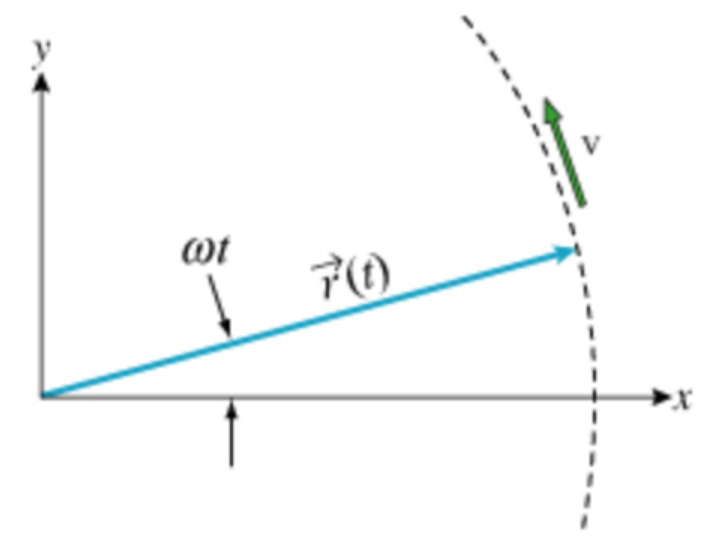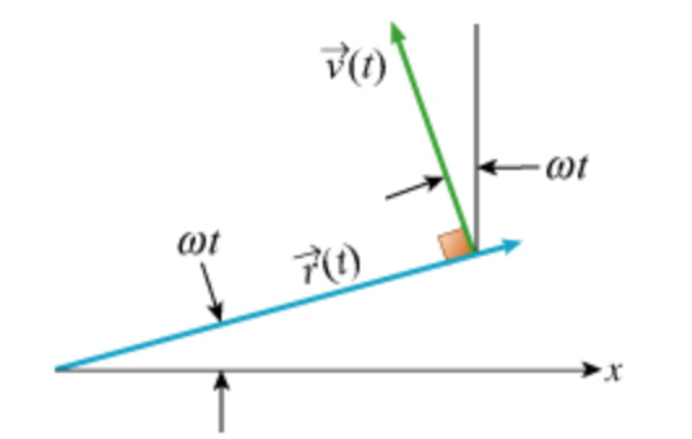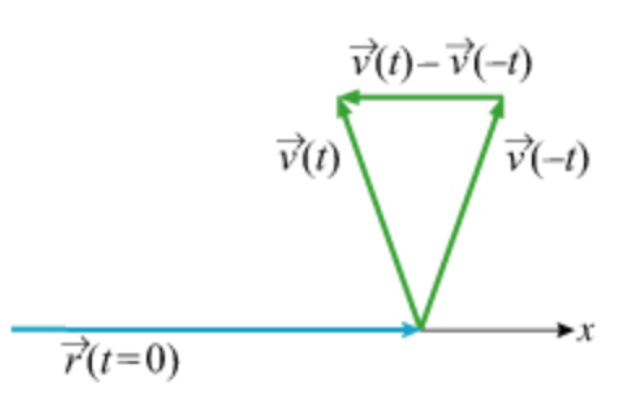# Problem: To understand that centripetal acceleration is the acceleration that causes motion in a circle. Acceleration is the time derivative of velocity. Because velocity is a vector, it can change in two ways: the length (magnitude) can change and/or the direction can change. The latter type of change has a special name, the centripetal acceleration. In this problem we consider a mass moving in a circle of radius R with angular velocity w,A) What is the velocity of the mass at a time t? You can work this out geometrically with the help of the hints, or by differentiating the expression for r (t) given in the introduction. (Figure 2) Express this velocity in terms of R, ω, t, and the unit vectors i^ and j^.B) What is the velocity of the mass at a time − t? Express this velocity in terms of R, ω, t, and the unit vectors i^ and j^.C) What is the average acceleration of the mass during the time interval from − t to t? (Figure 3) Express this acceleration in terms of R, ω, t, and the unit vectors i^ and j^.

###### FREE Expert Solution

A)

r(t) is given by:

r(t) = Rcos(ωt)i + Rsin(ωt)j

$\overline{){\mathbf{v}}{\mathbf{\left(}}{\mathbf{t}}{\mathbf{\right)}}{\mathbf{=}}\frac{\mathbf{d}\mathbf{r}\mathbf{\left(}\mathbf{t}\mathbf{\right)}}{\mathbf{d}\mathbf{t}}}$

So, $\begin{array}{rcl}\mathbf{v}\mathbf{\left(}\mathbf{t}\mathbf{\right)}& \mathbf{=}& \frac{\mathbf{d}}{\mathbf{d}\mathbf{t}}\mathbf{\left[}\mathbf{Rcos}\mathbf{\left(}\mathbf{\omega t}\mathbf{\right)}\mathbf{i}\mathbf{+}\mathbf{Rsin}\mathbf{\left(}\mathbf{\omega t}\mathbf{\right)}\mathbf{j}\mathbf{\right]}\end{array}$

82% (488 ratings)###### Problem Details

To understand that centripetal acceleration is the acceleration that causes motion in a circle. Acceleration is the time derivative of velocity. Because velocity is a vector, it can change in two ways: the length (magnitude) can change and/or the direction can change. The latter type of change has a special name, the centripetal acceleration. In this problem we consider a mass moving in a circle of radius R with angular velocity w,

A) What is the velocity of the mass at a time t? You can work this out geometrically with the help of the hints, or by differentiating the expression for r (t) given in the introduction. (Figure 2) Express this velocity in terms of R, ω, t, and the unit vectors i^ and j^.B) What is the velocity of the mass at a time − t? Express this velocity in terms of R, ω, t, and the unit vectors i^ and j^.C) What is the average acceleration of the mass during the time interval from − t to t? (Figure 3) Express this acceleration in terms of R, ω, t, and the unit vectors i^ and j^.